## Wednesday, April 29, 2020

### A DIY Binomial Cube/Trinomial Cube Manipulative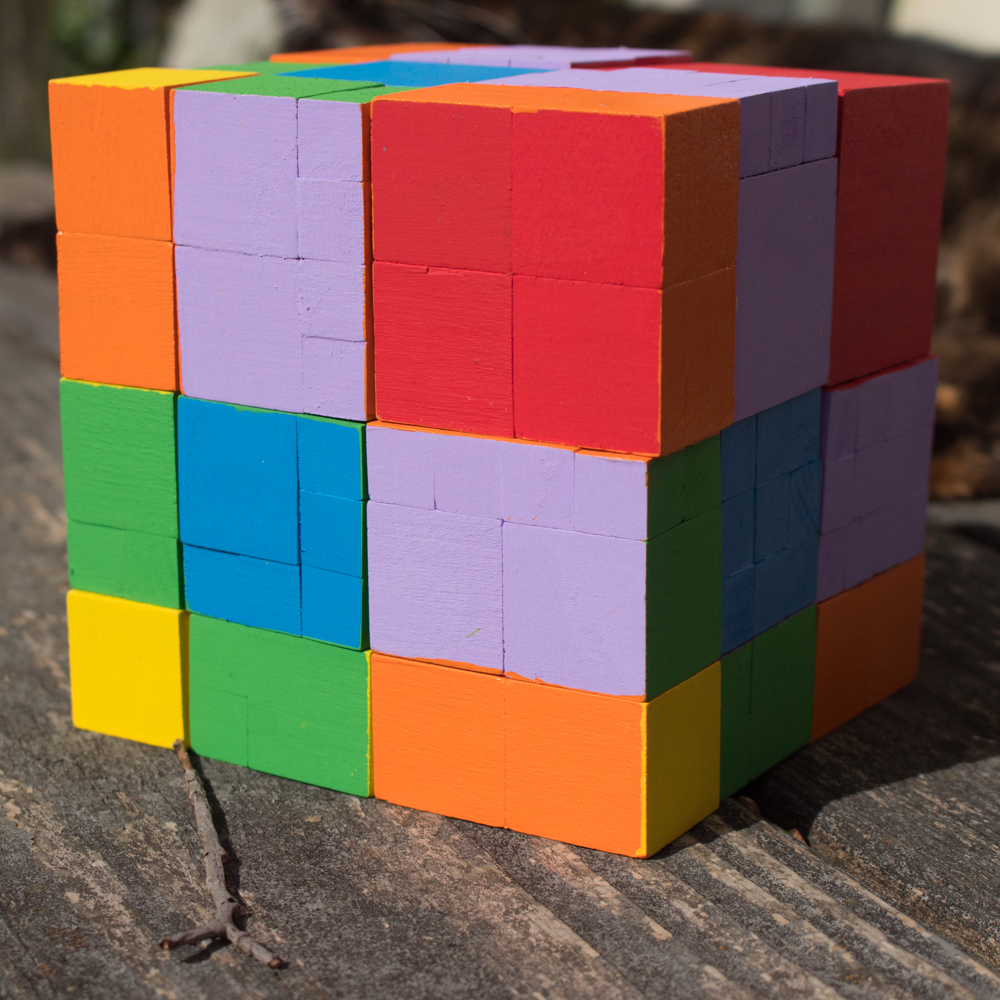If you've figured out that you can make a manipulative to literally represent (a + b + c)^2, then it's not a big stretch to figure out that you can also make a manipulative to literally represent (a + b + c)^3.

One is a literal square. The other is a literal cube!

I made this DIY trinomial cube to match faces with my DIY trinomial square. This means that it's also set to a 1" standard, NOT a 1 cm standard as are most of my other DIY math manipulatives. But since it was soooooo much easier to find 1", 1.5", and 2" blocks than it was to find 1 cm, 1.5 cm, and 2 cm blocks, it also means that I could take some major shortcuts on this build and save myself a lot of time gluing teeny-tiny wooden blocks together.

I'll take the shortcut every time!

The math isn't exactly tricky on this, but there's a lot of it, and a lot of moving parts, so bear with me.

The trinomial cube has three layers. Layer A is a trinomial square in which every prism has Height a. For my project, a = 1".

Layer B is a trinomial square in which every prism has Height b. For my project, b = 1.5".

Layer C is a trinomial square in which every prism has Height c. For my project, c = 2".

The base block for each layer is a wooden cube that I purchased from Casey's Wood Products:From left to right, that's a 2" cube, a 1.5" cube, and a 1" cube.

Let's go back to Layer A. We already know that every prism in Layer A will have a height of 1". The cube in Layer A is a^3, with a measurement of 1" x 1" x 1". This is nothing but a 1" wooden block, with no extra pieces added to it. As part of this layer you're also going to have a piece that's b^2, and a piece that's c^2, each with a height of 1", and the prisms that combine the measurements of ab, ac, and bc, each with a height of 1"

For every prism in this layer, you can start with a 1" wooden block, and glue on either .5" wooden blocks or 1" wooden blocks to make the correct sizes.

Here are all the prisms for Layer A:
• a^3 (1" x 1" x 1"). This is a 1" wooden block.
• ab^2 (1.5" x 1.5" x 1"). This is a 1" wooden block plus 10 .5" wooden blocks.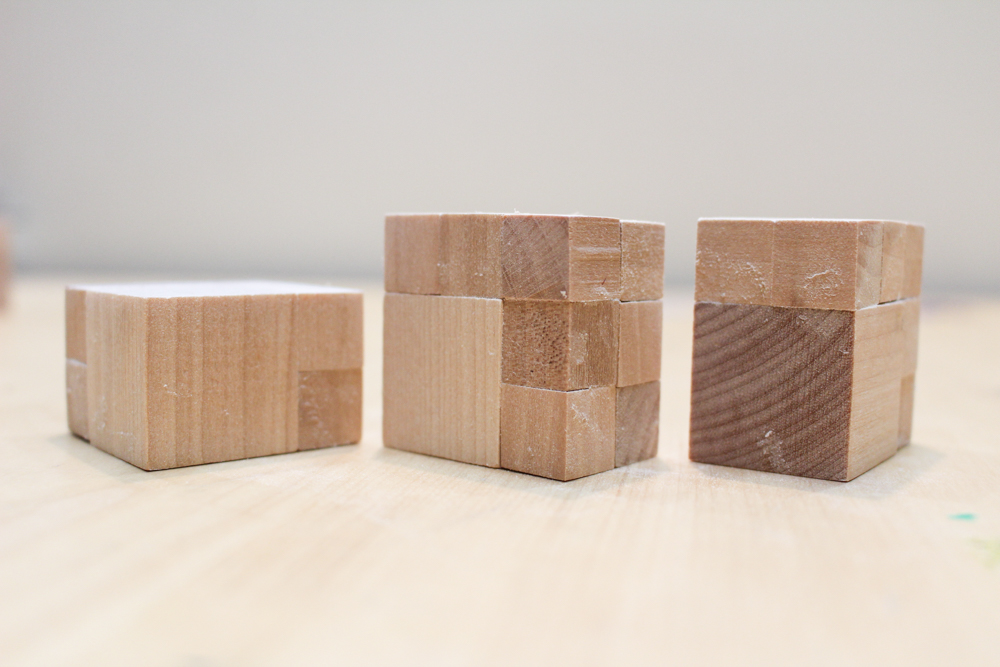You need one ab^2 for Layer A and two ab^2 for Layer B.
• ac^2 (2" x 2" x 1"). This is four 1" wooden blocks.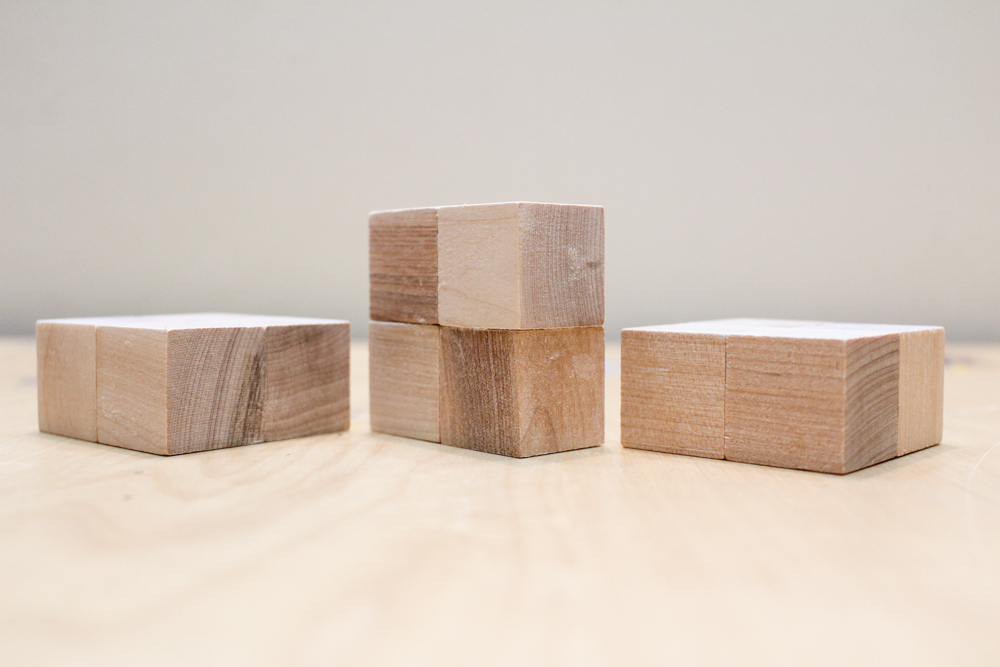You need one ac^2 for Layer A and two ac^2 for Layer C.
• two copies of  ba^2 (1.5" x 1" x 1"). This is a 1" wooden block plus four .5" wooden blocks.
• two copies of ca^2 (2" x 1" x 1"). This is two 1" wooden blocks.
• two copies of abc (1" x 1.5" x 2"). This is two 1" wooden blocks plus eight .5" wooden blocks.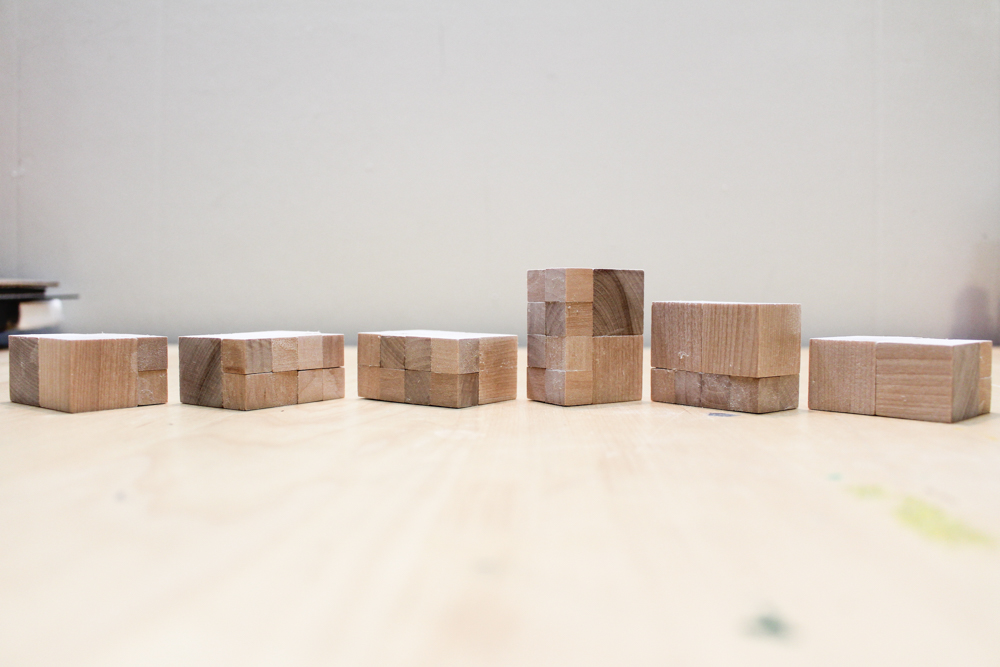You need two copies of abc for every layer, so six in total.

Every prism in Layer B will have a height of 1.5". The cube in Layer B is b^3, with a measurement of 1.5" x 1.5" x 1.5". This is nothing but a 1.5" wooden block, with no extra pieces added to it. As part of this layer you're also going to have a piece that's a^2, and a piece that's c^2, each with a height of 1.5", and the prisms that combine the measurements of ab, ac, and bc, each with a height of 1.5".

Here are all the prisms for Layer B:
• ba^2 (1.5" x 1" x 1"). This is a 1" wooden block plus four .5" wooden blocks.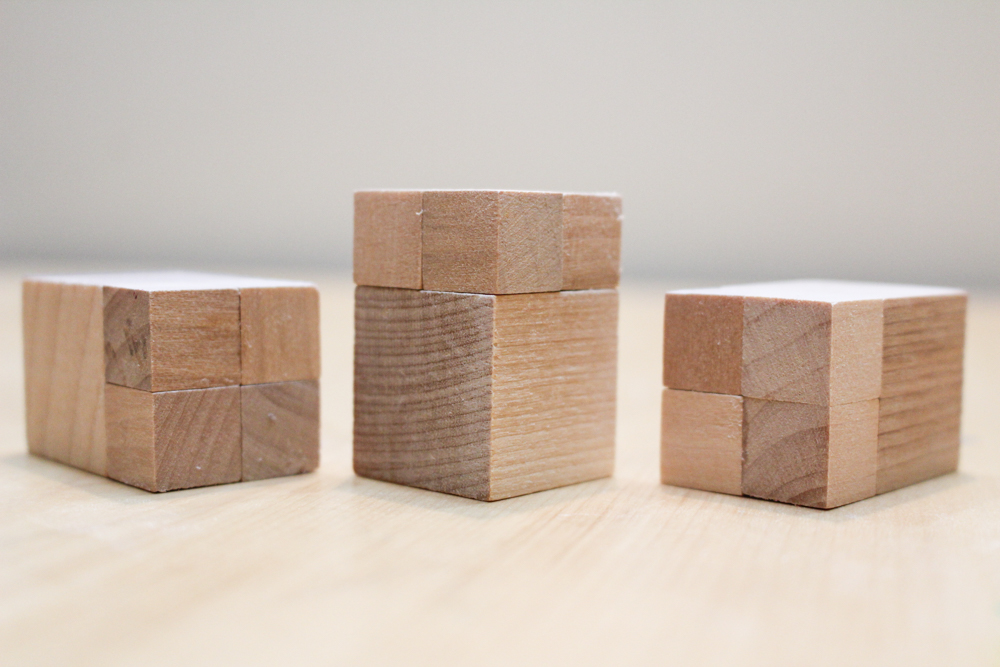You need two ba^2 for Layer A and one ba^2 for Layer B.
• b^3 (1.5" x 1.5" x 1.5"). This is a 1.5" wooden block.
• bc^2 (1.5" x 2" x 2"). This is a 1.5" wooden block plus twenty-one .5" wooden blocks.You need one bc^2 in Layer B and two bc^2 in Layer C.
• two copies of ab^2 (1.5" x 1.5" x 1"). This is a 1" wooden block plus 10 .5" wooden blocks.
• two copies of abc (1" x 1.5" x 2"). This is two 1" wooden blocks plus eight .5" wooden blocks.
• two copies of cb^2 (2" x 1.5" x 1.5"). This is a 1.5" wooden block plus nine .5" wooden blocks.You need two cb^2 for Layer B and one cb^2 for Layer C.

Every prism in Layer C will have a height of 2". The cube in Layer C is c^3, with a measurement of 2" x 2" x 2". This is nothing but a 2" wooden block, with no extra pieces added to it. As part of this layer you're also going to have a piece that's a^2, and a piece that's b^2, each with a height of 2", and the prisms that combine the measurements of ab, ac, and bc, each with a height of 2".

Here are all the prisms for Layer C:
• ca^2 (2" x 1" x 1"). This is two 1" wooden blocks.You need two ca^2 for Layer A and one ca^2 for Layer B.
• cb^2 (2" x 1.5" x 1.5"). This is a 1.5" wooden block plus nine .5" wooden blocks.
• c^3 (2" x 2" x 2"). This is a 2" wooden block.
• two copies of abc (1" x 1.5" x 2"). This is two 1" wooden blocks plus eight .5" wooden blocks.
• two copies of ac^2 (1" x 2" x 2"). This is four 1" wooden blocks.
• two copies of bc^2 (1.5" x 2" x 2"). This is a 1.5" wooden block plus twenty-one .5" wooden blocks.
I painted these prisms the same way I painted the trinomial square prisms, except for the cube, every face will be painted. All the 1"^2 faces are yellow, the 1"x1.5" faces are green----the 1.5"^2 faces are blue----the 1"x 2" faces are orange, the 1.5" x 2" faces are purple, and the 2"^2 faces are red:Some prisms in different layers are identical, so here are the total number of prisms by type that I needed:
• one a^3 (1" x 1" x 1"). This is a 1" wooden block.
• one b^3 (1.5" x 1.5" x 1.5"). This is a 1.5" wooden block.
• one c^3 (2" x 2" x 2"). This is a 2" wooden block.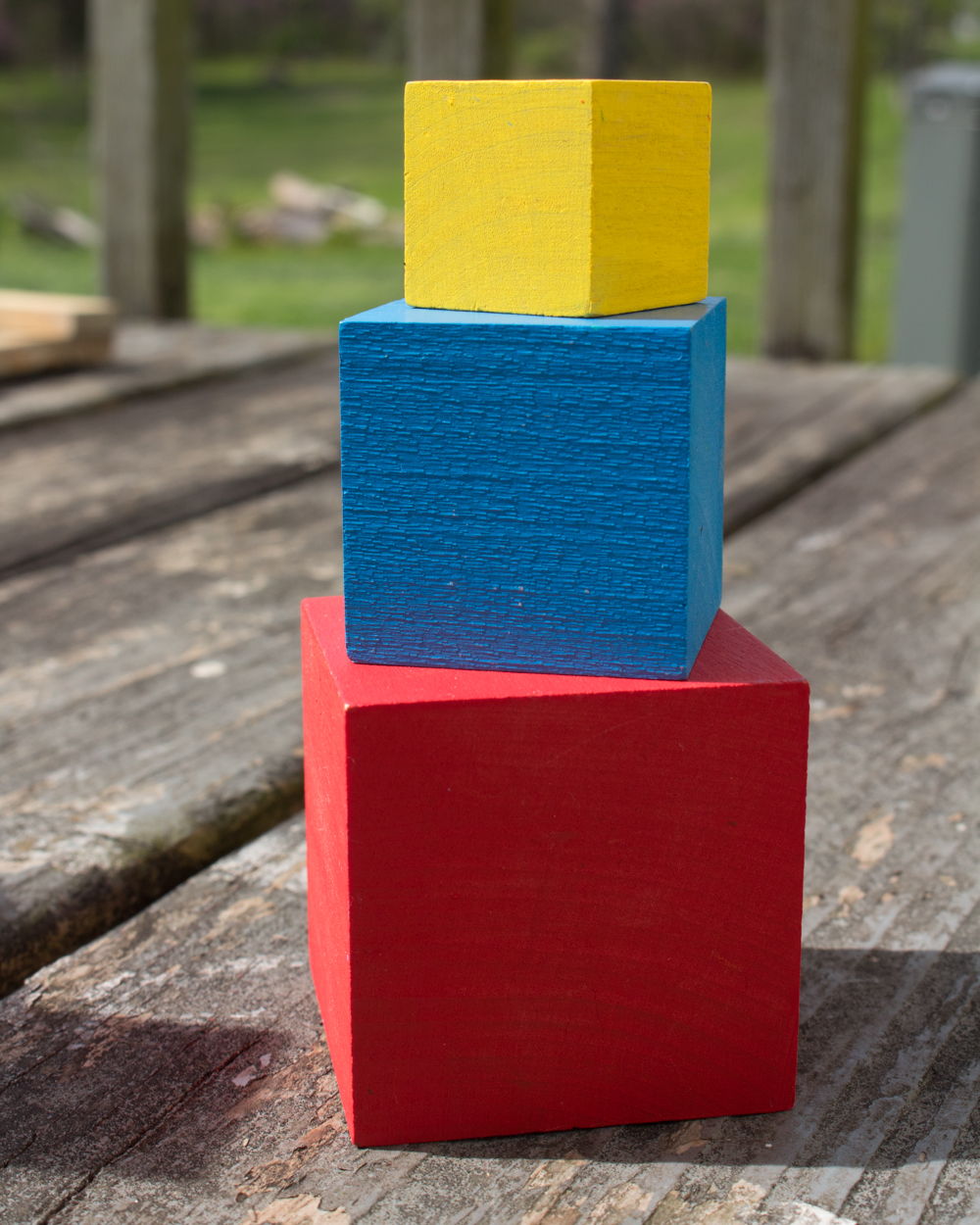Here's a^3, b^3, and c^3.
• three ba^2 (1.5" x 1" x 1"). This is a 1" wooden block plus four .5" wooden blocks.
• three ca^2 (2" x 1" x 1"). This is two 1" wooden blocks.
• three ab^2 (1.5" x 1.5" x 1"). This is a 1" wooden block plus 10 .5" wooden blocks.You need one ab^2 for Layer A and two ab^2 for Layer B.
• six abc (1" x 1.5" x 2"). This is two 1" wooden blocks plus eight .5" wooden blocks.you need two abc prisms for each layer.
There are a lot of abc blocks, so thankfully Spots was willing to help me photograph them!

• three cb^2 (2" x 1.5" x 1.5"). This is a 1.5" wooden block plus nine .5" wooden blocks.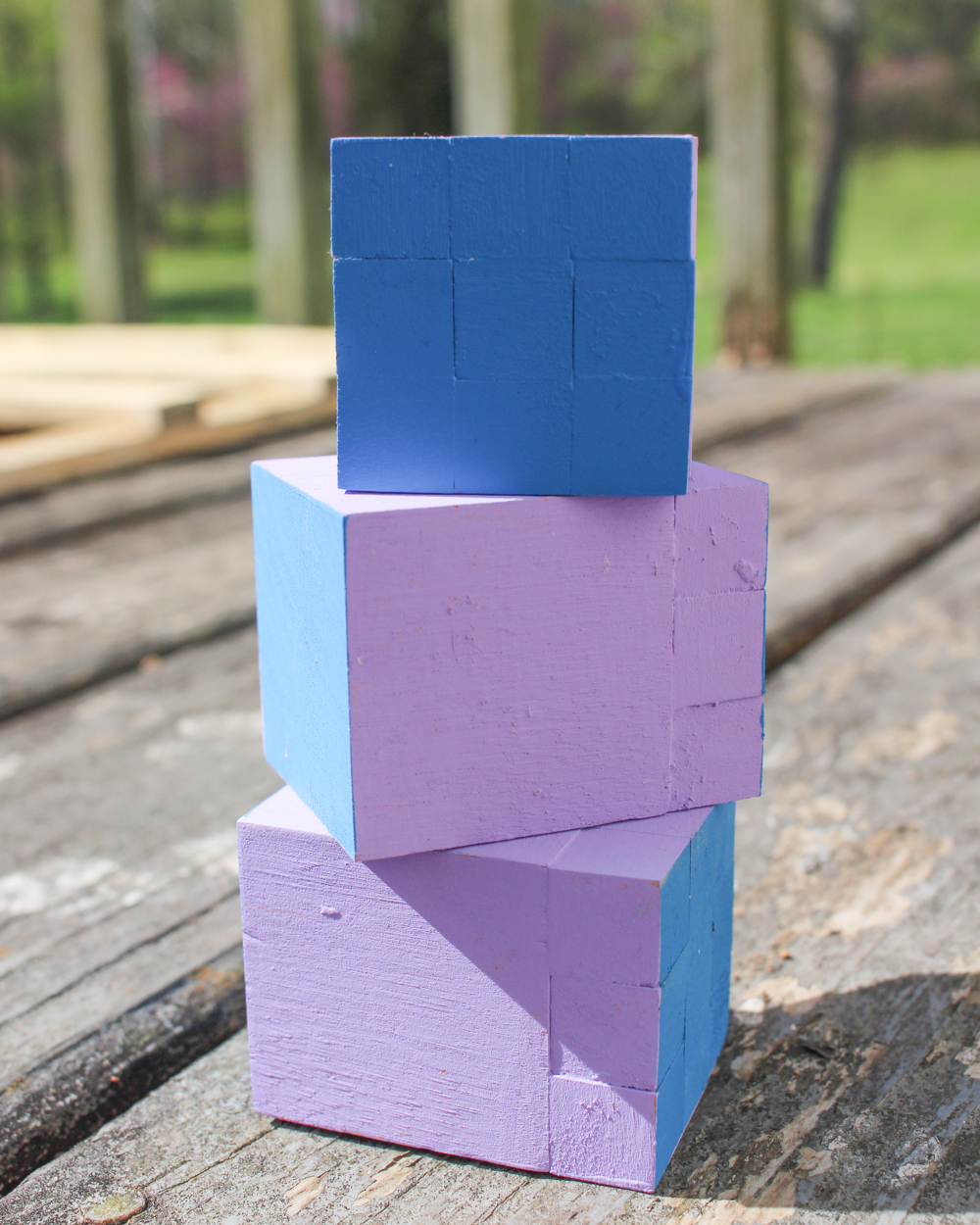three cb^2
• three ac^2 (1" x 2" x 2"). This is four 1" wooden blocks.three ac^2
• three bc^2 (1.5" x 2" x 2"). This is a 1.5" wooden block plus twenty-one .5" wooden blocks.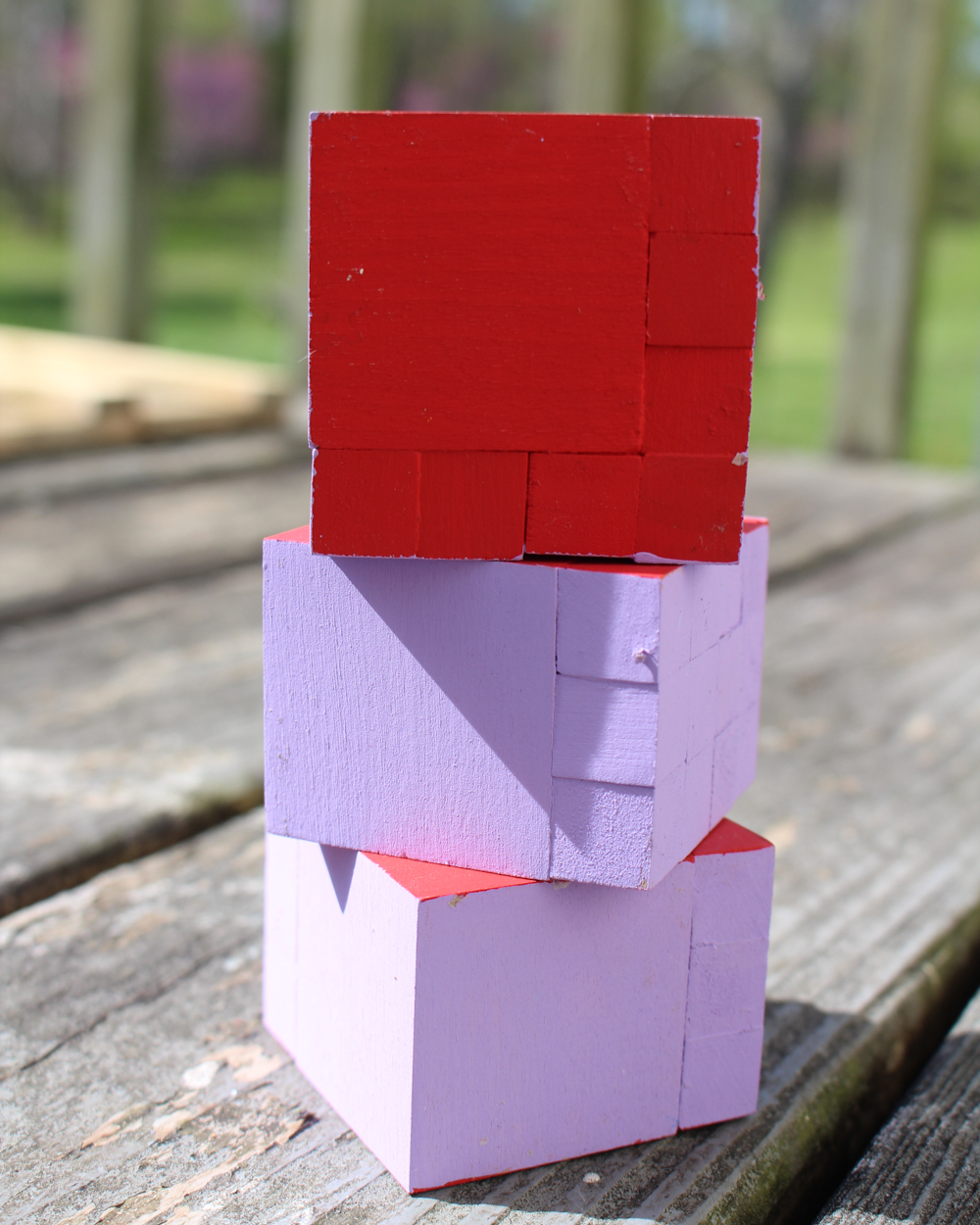three bc^2
Spots is sorry that she knocked over my blocks, so here's a blep to make up for it:

And also a boop for good measure:

And here's what it looks like when it's all finished!

### LAYER C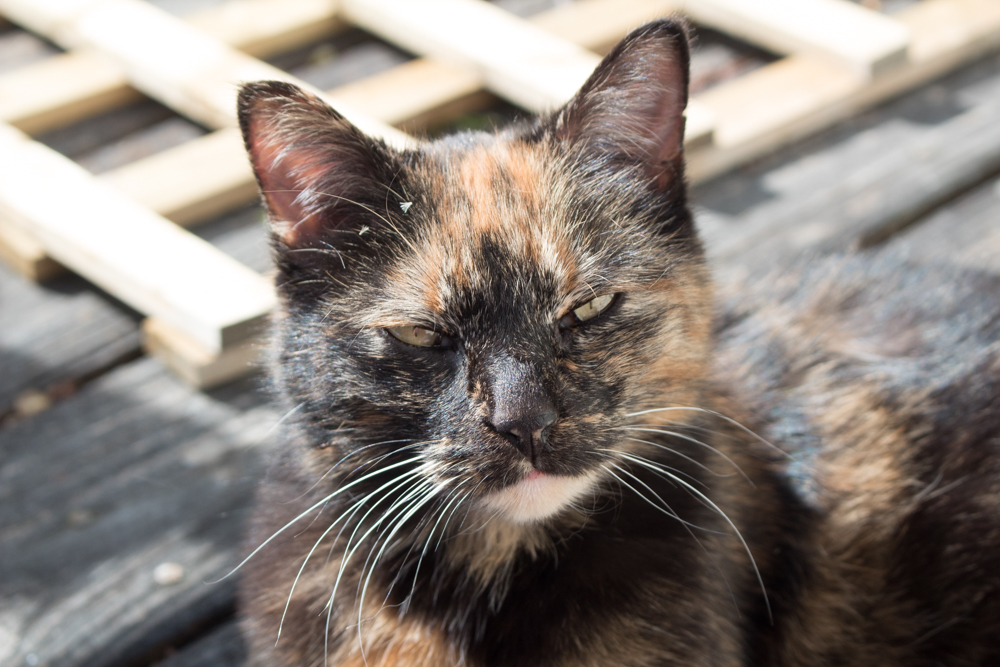Spots is still not helping...

The kids wandered over as I was finishing photographing the cube (meaning that now I had two teenagers AND a cat helping me...), so I told them this was a puzzle that I'd just finished building and invited them to see if they could put it together:

They didn't notice that it was a pattern, at first, and so made some unworkable choices:

Eventually, though, a light bulb came on...

And behold! It's laid out unusually, but it's our trinomial cube!

Here's the Montessori setup for the binomial and trinomial cubes. They're normally given to small children to work as a puzzle, which is a great way to make that higher level math familiar and not scary at all when they come to it later.

And here are a couple more references, if you want to plan a lesson around the binomial or trinomial cube:

Want to see what other mischief we (and the cats) manage to get up to with our brand-new DIY trinomial cube? Check out my Craft Knife Facebook page for updates!